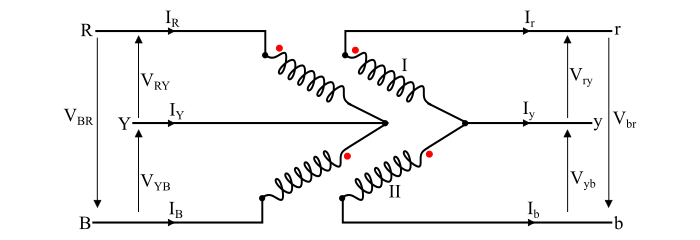# Open Delta Connection or V-V Connection of Transformers

If one transformer of delta-delta connected transformer bank is damaged or opened, then rest of the system will continue to supply the 3-phase power. If this damaged transformer is isolated, the remaining two transformers will function as a 3-phase bank with rating reduced to about 58 % that of the original delta-delta bank. This type of arrangement is known as open-delta or V-V connection.

Therefore, in the case of open-delta or V-V connection, two instead of three 1-phase transformers are used for three-phase operation.Now, let VRY, VYB and VBR be the applied voltages to the primary winding. The voltage induced in the secondary winding-I is Vry and the voltage induced in the secondary winding-II is Vyb. As, there is no winding between the points r and b, but there is a voltage between the points r and b. This voltage can be determined by applying KVL in the closed loop on the secondary side. Hence,

$$\mathrm{𝑉_{𝑟𝑦} + 𝑉_{𝑦𝑏} + 𝑉_{𝑏𝑟} = 0}$$

$$\mathrm{\Rightarrow 𝑉_{𝑏𝑟} = −𝑉_{𝑟𝑦} − 𝑉_{𝑦𝑏 }… (1)}$$

If VLP is the magnitude of line voltage on the primary side, then,

$$\mathrm{𝑉_{𝑅𝑌} = 𝑉_{𝐿𝑃}\angle0°}$$

$$\mathrm{𝑉_{𝑌𝐵} = 𝑉_{𝐿𝑃}\angle − 120°}$$

$$\mathrm{𝑉_{𝐵𝑅} = 𝑉_{𝐿𝑃}\angle + 120°}$$

If the leakage impedances of the transformers are neglected, then,

$$\mathrm{𝑉_{𝑟𝑦} = 𝑉_{𝐿𝑆}\angle0°}$$

$$\mathrm{𝑉_{𝑦𝑏} = 𝑉_{𝐿𝑆}\angle − 120°}$$

Where, VLS is the magnitude of the line voltage on the secondary side.

Now, by substituting the values of Vry and Vyb in the equation (1), we get,

$$\mathrm{𝑉_{𝑏𝑟} = −𝑉_{𝐿𝑆}\angle0° − 𝑉_{𝐿𝑆}\angle − 120°}$$

$$\mathrm{\Rightarrow\:𝑉_{𝑏𝑟} = (−𝑉_{𝐿𝑆} − 𝑗0) − (−0.5𝑉_{𝐿𝑆} − 𝑗0.866𝑉_{𝐿𝑆})}$$

$$\mathrm{\Rightarrow\:𝑉_{𝑏𝑟} = −0.5𝑉_{𝐿𝑆} + 𝑗0.866𝑉_{𝐿𝑆} = 𝑉_{𝐿𝑆}\angle + 120° … (2)}$$

From the eqn. (2), it can be seen that the voltage Vbr is equal in magnitude to the secondary line voltage and 120° displaced in time from the other two secondary voltages. Therefore, if the balanced 3-phase supply is connected to the primary windings of the open-delta connection, the balanced three-phase voltages are produced on the secondary sides, when the leakage impedances are negligible.

## Open-Delta Connection Calculations and Formulae

If V_{2} and I_{2} are the rated secondary voltage and rated secondary current respectively of the delta-delta connected transformer. Then, the line current to the load of a delta connected system is √3𝐼_{2}. Therefore, the normal delta load VA is,

$$\mathrm{𝑆_{\triangle−\triangle} = √3 × line\:voltage × line\:current}$$

$$\mathrm{\Rightarrow\:𝑆_{\triangle−\triangle} = √3 × 𝑉_{2}× (√3𝐼_{2}) = 3𝑉_{2}𝐼_{2} … (3)}$$

Now, one transformer is removed, the delta-delta connection becomes open-delta connection and the lines are in series with the windings of the transformer. Thus, the secondary line currents is equal to the rated secondary current. Therefore, the load VA carried by the opendelta connection without exceeding the ratings of the transformer is,

$$\mathrm{𝑆_{V−V} = √3 𝑉_{2} 𝐼_{2} … (4)}$$

Therefore,

$$\mathrm{\frac{𝑆_{V−V}}{𝑆_{\triangle−\triangle}}=\frac{√3 𝑉_{2} 𝐼_{2}}{3 𝑉_{2} 𝐼_{2}}=\frac{1}{√3}= 0.577}$$

$$\mathrm{\Rightarrow S_{V-V}=57.7\% \:of\:S_{\triangle-\triangle}\:\:\:\:...(5)}$$

Hence, the load that can be carried by an open-delta transformer without exceeding the ratings of it is 57.7 % of the original load that is carried by the normal delta-delta transformer bank.

Also,

$$\mathrm{\frac{VA\:per\:transformer}{Total\:3 − phase\:VA}=\frac{𝑉_{2} 𝐼_{2}}{√3 𝑉_{2} 𝐼_{2}}=\frac{1}{√3} = 0.577… (6)}$$

From the eq. (6), it is clear that the VA supplied by each transformer in an open-delta system is also 57.7 % of the total 3-phase VA.

Now, if three 1-phase transformers are connected in delta-delta fashion and supplying rated load. As soon as it becomes an open-delta transformer, the current in each winding is increased by √3 times, i.e., full line current flows in each of the remaining two phase windings of the transformer. Hence, each transformer in the open-delta system is overloaded by 73.2%. Therefore, it is an important precaution that the load should be reduced by √3 times in the case of V-V connection of the transformers. Otherwise, the remaining two transformers may breakdown due to overheating.

## Power Supplied by Open-Delta (V-V) Connected Transformer

When an open-delta bank of two transformers supplies a balanced three phase load of power factor cos φ, then the phase angle between the line voltage and the line current in one transformer is (30°+φ) whereas the phase angle between the line voltage and the line current in the other transformer is (30°-φ). Hence, one transformer operates at a power factor of cos(30°+φ) and the other at cos(30°-φ). Therefore, the power supplied by the transformers is given by,

$$\mathrm{𝑃_{1} = 𝑉_{𝐿} 𝐼_{𝐿}\:cos(30° + φ)}$$

$$\mathrm{𝑃_{2} = 𝑉_{𝐿} 𝐼_{𝐿}\:cos(30° - φ)}$$

The total power supplied by the transformers is

$$\mathrm{𝑃 = 𝑃_{1} + 𝑃_{2} = 𝑉_{𝐿} 𝐼_{𝐿 }cos(30° + φ) + 𝑉_{𝐿} 𝐼_{𝐿}\:cos(30° − φ)}$$

$$\mathrm{\Rightarrow\: 𝑃 = 𝑉_{𝐿} 𝐼_{𝐿} (cos\:30°\:cos\:φ − sin\:30°\:sin φ + cos \:30°\:cos\:φ + sin 30°\:sin φ)}$$

$$\mathrm{\Rightarrow\: 𝑃 = 2 𝑉_{𝐿} 𝐼_{𝐿}\:cos \:30°\:cos\:φ}$$

$$\mathrm{\Rightarrow 𝑃 = √3 𝑉_{𝐿} 𝐼_{𝐿 }cos\:φ … (7)}$$

At the load of unity power factor i.e.

$$\mathrm{cos φ = 1 \Rightarrow\: φ = 0°}$$

Hence, the power supplied by each transformer is

$$\mathrm{𝑃_{1} = 𝑃_{2} = 𝑉_{𝐿} 𝐼_{𝐿}\:cos 30° =\frac{√3}{2}𝑉_{𝐿} 𝐼_{𝐿} … (8)}$$

## Applications of the Open Delta or V-V Connection

Following are the applications in which open-delta system is used −

• As a temporary measure, when one transformer of a delta-delta bank is damaged and removed for maintenance.

• The V-V connected transformers are used to supply a combination of large 1-phase and smaller 3-phase loads.

• The V-V connected transformers are used to provide service in a new development area where the full growth of load requires several years. In such cases, an open-delta system is installed in the initial stage and whenever the need arises at a future date to accommodate the growth in the power demand, a third transformer is added for deltadelta operation. This third transformer increases the capacity of the bank by 73.2%.

Updated on: 13-Aug-2021

4K+ Views